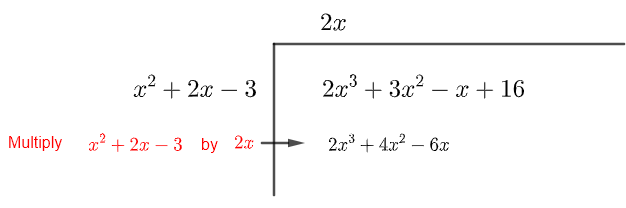# Polynomial Division

## Polynomial Division Definition

 The division of polynomial $P(x)$ by the polynomial $S(x)$ may be written as follows 
$\dfrac{P(x)}{S(x)} = Q(x) + \dfrac{R(x)}{S(x)} \qquad (I)$
where $Q(x)$ is the quotient and $R(x)$ is the remainder of the division.
If we multiply all terms in (I) above by $S(x)$, we may write the division as
$P(x) = Q(x) S(x) + R(x)$

To divide a polynomial , we make use of the long division process. Before bginning the long division process, write the dividend and the divisor in descending powers of x. In order to help align like terms, use 0 as the coefficient of any missing power.
A video on long division of polynomials is included.
An online polynomial division calculator, that may be used to check results of division done by hand, is included

## Example Polynomial Division

Example 1:
Divide
$\dfrac{2x^3 + 3x^2 - x + 16}{x^2 + 2x - 3}$

Solution:
The dividend $2x^3 + 3x^2 - x + 16$ is a polynomial of degree 3. The divisor $\; x^2 + 2x - 3 \;$ is a polynomial of degree $2$. By laws of the exponents, we expect that the quotient is a polynomial of degree $1$ or $0$.
STEP 1: We first divide the term with highest power in the dividend $\; 2x^3 \;$ by the term with the highest power in the divisor $\; x^2 \;$to obtain a quotient equal to $\; 2x \;$ and organize all three terms as follows.STEP 2: We next multiply the divisor $\; x^2 + 2x - 3 \;$ by the quotient $\; 2x \;$and organize the result as followsSTEP 3: We next subtract the result of the multiplication from the dividend as followsSTEP 4: We now divide the term with the highest power in the subtraction result $\; -x^2 \;$ by the term with the highest power in the divisor $\; x^2 \;$ to obtain $\; -1 \;$ and organize all terms as followsSTEP 5: We next multiply the divisor $\; x^2 + 2x - 3 \;$ by $\; -1 \;$ and organize all terms as followsSTEP 6: Subtract the result of the last multiplication from the term before it and organize the results as follows.We now stop the process since the last term $\; 7x + 13 \;$ has a degree smaller that that of the divisor $\; x^2 + 2x - 3 \;$.

The result of the long division may be written as follows
$\dfrac{2x^3 + 3x^2 - x + 16}{x^2 + 2x - 3} = 2x - 1 + \dfrac{7x + 13}{x^2 + 2x - 3}$
or also as follows
$2x^3 + 3x^2 - x + 16 = (2x - 1)(x^2 + 2x - 3) + 7x + 13$
Vocabulary associated with the long division process
$2x^3 + 3x^2 - x + 16$ is the dividend
$x^2 + 2x - 3$ is the divisor
$2x - 1$ is the quotient
$7x + 13$ is the remainder

## More References and Links to Polynomials

Algebra and Trigonometry - Swokowsky Cole - 1997 - ISBN: 0-534-95308-5
Algebra and Trigonometry with Analytic Geometry - R.E.Larson , R.P. Hostetler , B.H. Edwards, D.E. Heyd - 1997 - ISBN: 0-669-41723-8
polynomial Functions.